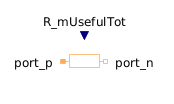Total Harmonic Distortion - MapleSim Help

Total Harmonic Distortion

Output the total harmonic distortion (THD)Description The Total Harmonic Distortion component determines the total harmonic distortion (THD) over the given period $\frac{1}{f}$. Consider that the input $u$ consists of harmonic RMS components ${U}_{1}$, ${U}_{2}>$, ${U}_{3}$, etc. The total RMS component is then determined by:The calculation of the total harmonic distortion is based on the parameter $\mathrm{useFirstHarmonic}$. The default value $\mathrm{useFirstHarmonic}=\mathrm{true}$ represents the standard THD calculation used in electrical engineering http://www.electropedia.org/iev/iev.nsf/display?openform&ievref=551-20-13 . The non-default value $\mathrm{useFirstHarmonic}=\mathrm{false}$ calculates the THD typically used for the assessment of audio signals. If $\mathrm{useFirstHarmonic}=\mathrm{true}$, the total higher harmonic content (harmonic order numbers > 1) refers to the RMS value of the fundamental wave:If $\mathrm{useFirstHarmonic}=\mathrm{false}$, the total higher harmonic content (harmonic order numbers > 1) refers to the total RMS:In case of a zero input signal or within the first period of calculation, the boolean output signal $\mathrm{valid}$ becomes $\mathrm{false}$ to indicate that the calculation result is not valid. Valid calculations are indicated by $\mathrm{valid}=\mathrm{true}$.Connections

 Name Description Modelica ID $u$ Real input signal u $y$ Real output signal y $\mathrm{valid}$ Boolean output signal; true, if output y is valid validParameters

 Name Default Units Description Modelica ID $f$ $\mathrm{Hz}$ Base frequency f use first harmonic $\mathrm{true}$ THD with respect to first harmonic, if true; otherwise with respect to total RMS useFirstHarmonicModelica Standard Library The component described in this topic is from the Modelica Standard Library. To view the original documentation, which includes author and copyright information, click here.